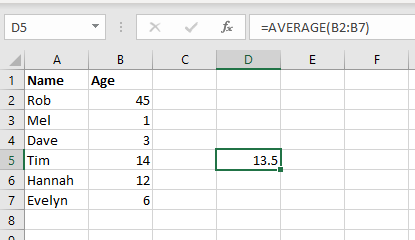# Help With Excel – Learn The Basics

Getting some basic functions help with Excel never goes astray. When you learn the benefit spreadsheets can provide, you can boost your productivity and organisation skills tremendously. From keep track of business expenses, sales and even a share houses’ budget, Excel is a great help.

Here’s Monopoly inside Excel!

Excel complexities can be tremendous, there have been programmers that have made entire 3D games in the spreadsheet so if you feel the need to get more advanced help you could find a detailed course here: Udemy Excel Courses.

But read along to get basic help with Excel below.

## Excel Sum Formula

The sum Formula in Excel allows you to add multiple cells to have the result appear in the cell where you enter the formula.

Multiplication, subtraction and division can be used with this Excel formula too. Make sure you put brackets around groups of calculations to set the right order of calculations though.

This is cell B2 plus cell B5. You need to put an equal sign before the formula to let Excel know that you intend to write a formula.

Here’s what it looks like in Excel, with some more complex examples too.

## Help With Excel – The Excel Sort Function

Sort a number of rows alphabetically or numerically with the Excel sort function tool.

Find the sort feature under the ‘Data’ tab at the top navigation. It’s usually easiest to press the Sort button.

Manually configure which column you want to sort by, as well as the order that it’s sorted in. The AZ buttons attempt to guess what you intend to sort by which often isn’t accurate.

When you use the sort function, be sure to highlight all the columns that you intend to sort, as well as their titles.

## Excel Column Sum

Use the SUM feature to be more efficient with your summaries. This is a good formula for subtotals and totals of columns for example.

The formula looks like this: =SUM(B2:B7). It starts with the equals sign as usual, and then gets the highest cell to add, then the lowest. It adds all cells in between also.

The AutoSum button in the top toolbar is also available. Highlight the columns you want to sum up and when you press the AutoSum button it will put the result under the last number that you’ve highlighted.

Here’s a little video showing how to use the Sum and AutoSum features:

## Excel Average Function

There are statistical functions in Excel too. This function is very similar to the Excel sum formula, but gets an average instead of total. Here’s what getting the average of some cells looks like:The Excel average formula grabs the chosen sections of column. So in the above image, we’ve told it to get an average of the numbers in the B column, between rows 2 and 7. This is shown by using the formula =AVERAGE(B2:B7). The result is shown in the column that the formula is written, in this example that is D5.

Here it is in realtime:

## Excel Features: Appearance, Freezing Rows

Excel is very much like word when formatting text. You can bold and colour text but you can also colour cells. This helps with defining titles and segments of your spreadsheets.

Another handy function is freezing rows. If you have a long spreadsheet and want to always see the names of columns, you can freeze the top row so it moves down the page when you scroll down.

Here’s some simple formatting tips to use in Excel

## More Excel Help – Spreadsheet Templates

Now that you’ve got some basic of Excel formulas down, you’ll be able to utilise our spreadsheet templates more effectively.

You can get a lot out of our accounting and sales spreadsheets, especially when you can customise the formulas when necessary.